Home

# Flux expulsion with dynamics

## Abstract

In the process of flux expulsion, a magnetic field is expelled from a region of closed streamlines on a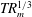$TR_{m}^{1/3}$ time scale, for magnetic Reynolds number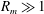$R_{m}\gg 1$ (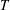$T$ being the turnover time of the flow). This classic result applies in the kinematic regime where the flow field is specified independently of the magnetic field. A weak magnetic ‘core’ is left at the centre of a closed region of streamlines, and this decays exponentially on the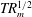$TR_{m}^{1/2}$ time scale. The present paper extends these results to the dynamical regime, where there is competition between the process of flux expulsion and the Lorentz force, which suppresses the differential rotation. This competition is studied using a quasi-linear model in which the flow is constrained to be axisymmetric. The magnetic Prandtl number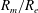$R_{m}/R_{e}$ is taken to be small, with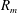$R_{m}$ large, and a range of initial field strengths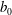$b_{0}$ is considered. Two scaling laws are proposed and confirmed numerically. For initial magnetic fields below the threshold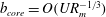$b_{core}=O(UR_{m}^{-1/3})$ , flux expulsion operates despite the Lorentz force, cutting through field lines to result in the formation of a central core of magnetic field. Here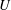$U$ is a velocity scale of the flow and magnetic fields are measured in Alfvén units. For larger initial fields the Lorentz force is dominant and the flow creates Alfvén waves that propagate away. The second threshold is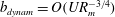$b_{dynam}=O(UR_{m}^{-3/4})$ , below which the field follows the kinematic evolution and decays rapidly. Between these two thresholds the magnetic field is strong enough to suppress differential rotation, leaving a magnetically controlled core spinning in solid body motion, which then decays slowly on a time scale of order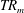$TR_{m}$ .

## References

Hide All
MathJax
MathJax is a JavaScript display engine for mathematics. For more information see http://www.mathjax.org.

# Flux expulsion with dynamics

## Metrics

### Full text viewsFull text views reflects the number of PDF downloads, PDFs sent to Google Drive, Dropbox and Kindle and HTML full text views.

Total number of HTML views: 0
Total number of PDF views: 0 *Loading metrics...

### Abstract viewsAbstract views reflect the number of visits to the article landing page.

Total abstract views: 0 *Loading metrics...

* Views captured on Cambridge Core between <date>. This data will be updated every 24 hours.

Usage data cannot currently be displayed.